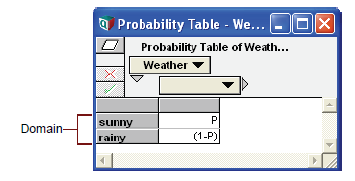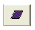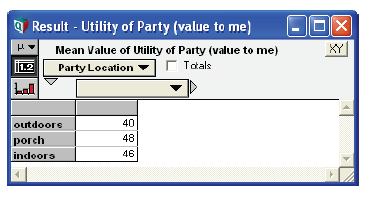# Custom discrete probabilities

(diff) ← Older revision | Latest revision (diff) | Newer revision → (diff)

Release: 4.6  •  5.0  •  5.1  •  5.2  •  5.3  •  5.4  •  6.0  •  6.1  •  6.2  •  6.3  •  6.4

These functions let you specify a discrete probability distribution using a custom set of values, text (label) values, or numbers.

## ProbTable(): Probability Tables

To describe a probability distribution on a discrete variable whose domain is a list of numbers or list of labels, you use special kind of edit table called a probability table (or probTable), see Arrays and Indexes and ProbTable.

Create a probability table: To define a variable using a probability table:

1. Determine the variable’s domain — number or labels for its possible values.
2. Select the variable and view its definition attribute in the Object window or Attribute panel of the Diagram window.
3. Press the expr menu above the definition field and select Probability Table.If the variable already has a definition, it confirms that you wish to replace it.
Tip
If the definition of a variable is already a probability table, a ProbTable button appears in the definition. Click it to open the Edit Table window (see Defining a variable as an edit table).
4. The Indexes dialog opens to confirm your choices for the indexes of the table. It already includes the selected variable (Self) among the selected indexes. Other options are variables with a domain that is a list of numbers or list of labels. Add or remove any other variables that you want to condition this variable on.Tip
Self is required as an index of a probability table. It refers to the domain (possible values) of this variable.
5. Click the OK button. An Edit Table window appears.
6. Enter the possible values for the domain in the left column. As in any edit table, press Enter or down-arrow in the last row to add a row. Select Insert row (Control+i) or Delete row (Control+k) from the Edit menu. If they are numbers, they must be in increasing order.
7. Enter the probability of each possible outcome in the second column. The probabilities should sum to 1. You may enter literal numbers or expressions.

Example: If P is a variable whose value is a probability (between 0 and 1) and the possible weather outcomes are sunny and rainy, you might define a probability table for weather like this.Expression view of probability table: The Weather probability table when viewed as an expression, looks like this.

ProbTable(Self)(P, (1 - P))

The domain values do not appear in the expression view, and it is not very convenient for defining a probability table. More generally, the expression view of a multidimensional probability table looks like this:

ProbTable(i1, i2, … in) (p1, p2, p3, … pm)

This example is an «n»-dimensional conditional probability table, indexed by the indexes «i1», «i2», … «in». One index must be Self. «p1», «p2», «p3», … «pm» are the probabilities in the array. «m» is the product of the sizes of the indexes «i1», «i2», … «in».

Add a conditioning variable: You might wish to add one or more conditioning variables to a probability table, to create conditional dependency. Each discrete conditioning variable adds a dimension to the table. For example, in the Weather probability table, shown above, the probability of rain might depend on the season. So you might have Season as a conditioning variable, defined as a list of labels:

Variable Season := ['Winter', 'Spring', 'Summer', 'Fall']
1. Open the Edit Table window by clicking the ProbTable button.
2. Click the indexesbutton to open the Indexes dialog.
3. Click the All Variables checkbox above the left hand list.
4. Move the desired variable, e.g., Season, to add it as an index.
5. Click OK to accept the changes.

The resulting table is indexed by both the domain of your variable and the domains of the conditionally dependent variables. You need to enter a probability for each cell. The probabilities must sum to one over the domain of the variable (sunny and rainy in the example), not over the conditioning index(es).

Tip
You must have already specified the variables as probability tables, before adding them with the Indexes dialog.

## DetermTable(): Deterministic conditional table

DetermTable() defines the value of a variable as a deterministic (not uncertain) function of one or more discrete variables. It gives a value conditional on the value of one or more discrete variables, often including a probabilistic discrete variable and a discrete decision variable defined as a list. DetermTable() is described in Array functions, but we also include it in this section on discrete probability distributions, even though it is not probabilistic, because you usually use it in conjunction with ProbTable and other discrete distributions. It is an editable table, like ProbTable, but with a single (deterministic) value, number, or text, in each cell.

The DetermTable() function looks like an edit table or a probability table, with an index (dimension) from each discrete variable on which it depends. Unlike ProbTable(), it does not need a self index. Its result is probabilistic if any of its conditioning variables are probabilistic.

For the steps to create a determTable, see Creating a DetermTable.

Example: In Create a probability table above, Weather is defined as a probability table. If P, the probability of “sunny” is 0.4, then the probability of “rainy” is 0.6. Party_location is a decision variable with values ['outdoors', 'porch','indoors']. value_to_me is a determTable, containing utility values (or “payoffs”) for each combination of Party_location and Weather.Evaluating value_to_me gives the value of each party location, considering the uncertain distribution of Weather. The mean value of value_to_me is the expected utility.## ChanceDist(p, a, i)

Creates a discrete probability distribution, where «a» is an array of outcome values, numbers or text, and «p» is the corresponding array of probabilities. «a» and «p» must both be indexed by «i».

When to use: Use: ChanceDist instead of ProbTable when:

• The array of outcome «a» is multidimensional.
• You want a distribution where each outcome in «a» is equally likely. In this case, you can specify «p» as 1.

or

• You want to use other variables or expressions to define the outcomes or probability arrays.

Example:

Index Index_b :=
 Red White Blue
Variable Array_q :=
Index_b ▶
Red White Blue
0.3 0.2 0.5

The domain of the variable is a list of labels: ['Red', 'White', 'Blue'].

ChanceDist(Array_q, Index_b, Index_b) →